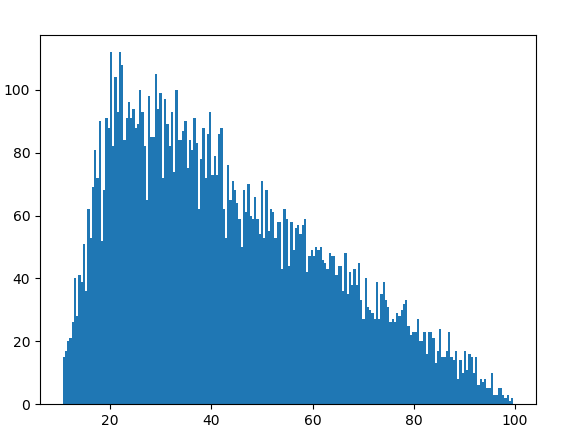# random.triangular() method in Python

`triangular()` is an inbuilt method of the `random` module. It is used to return a random floating point number within a range with a bias towards one extreme.

Syntax : random.triangular(low, high, mode)

Parameters :
low : the lower limit of the random number
high : the upper limit of the random number
mode : additional bias; low < mode < high

if the parameters are (10, 100, 20) then due to the bias, most of the random numbers generated will be closer to 10 as opposed to 100.

Returns : a random floating number

Example 1:

 `# import the random module ` `import` `random ` ` `  `# determining the values of the parameters ` `low ``=` `10` `high ``=` `100` `mode ``=` `20` ` `  `# using the triangular() method ` `print``(random.triangular(low, high, mode)) `

Output :

`22.614510550239572`

Example 2: If we generate the number multiple times we can probably identify the bias.

 `# import the random module ` `import` `random ` ` `  `# determining the values of the parameters ` `low ``=` `10` `high ``=` `100` `mode ``=` `20` ` `  `# running the triangular method with the ` `# same parameters multiple times ` `for` `i ``in` `range``(``10``): ` `    ``print``(random.triangular(low, high, mode)) `

Output :

```58.645768016894735
46.690692250503226
33.57590419190895
52.331804090351305
33.09451214875767
12.03845752596168
32.816080679206294
20.4739124559502
82.49208123077557
63.511062284733015```

Example 3: We can visualize the triangular pattern by plotting a graph.

 `# import the required libraries ` `import` `random ` `import` `matplotlib.pyplot as plt ` ` `  ` `  `# store the random numbers in a list ` `nums ``=` `[] ` `low ``=` `10` `high ``=` `100` `mode ``=` `20` ` `  `for` `i ``in` `range``(``10000``): ` `    ``temp ``=` `random.triangular(low, high, mode) ` `    ``nums.append(temp) ` `     `  `# plotting a graph ` `plt.hist(nums, bins ``=` `200``) ` `plt.show() `

Output :My Personal Notes arrow_drop_upCheck out this Author's contributed articles.

If you like GeeksforGeeks and would like to contribute, you can also write an article using contribute.geeksforgeeks.org or mail your article to contribute@geeksforgeeks.org. See your article appearing on the GeeksforGeeks main page and help other Geeks.

Please Improve this article if you find anything incorrect by clicking on the "Improve Article" button below.

Article Tags :

Be the First to upvote.

Please write to us at contribute@geeksforgeeks.org to report any issue with the above content.